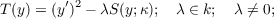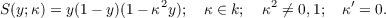#### Vol. 74, No. 1, 1978

 Recent Issues Vol. 325: 1 Vol. 324: 1  2 Vol. 323: 1  2 Vol. 322: 1  2 Vol. 321: 1  2 Vol. 320: 1  2 Vol. 319: 1  2 Vol. 318: 1  2Online Archive Volume: Issue:The Journal Subscriptions Editorial Board Officers Contacts Submission Guidelines Submission Form Policies for Authors ISSN: 1945-5844 (e-only) ISSN: 0030-8730 (print) Special Issues Author Index To Appear Other MSP Journals
Transcendental constants over the coefficient fields in differential elliptic function fields

### Keiji Nishioka

Vol. 74 (1978), No. 1, 191–197
##### Abstract

Let k be a differential field of characteristic 0, and Ω be a universal extension of k. Suppose that the field of constants k0 of k is algebraically closed. Consider the following dlfferential polynomial of the first order over k in a single indeterminate y:hereTake a generic point z of the general solution of T. Then, z is transcendental over k, and k(z,z) is called a differential elliptic function field.

We prove the following:

Theorem. Let k(z,z) be a differential elliptic function field over k. Then, there exists a finitely generated differential extension field k of k such that the following three conditions are satisfied:

1. z is transcendental over k;
2. the field of constants of k is the same as k0;
3. there exists an element ζ of Ω such that k(z,z) = k(ζ,ζ) and (ζ)2 = 4S(ζ;κ) with the same modulus as κ.

Primary: 12H05
Secondary: 34A20
##### Milestones